## Apparent Power and Power Factor:

Apparent Power – The power factor is useful in determining useful power (true power) transferred to a load. The highest power factor is 1, which indicates that the current to a load is in phase with the voltage across it (i.e. in the case of resistive load). When the power factor is 0, the current to a load is 90° out of phase with the voltage (i.e. in case of reactive load).

Consider the following equation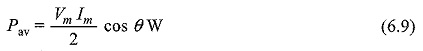In terms of effective values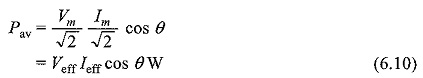The average power is expressed in watts. It means the useful power transferred from the source to the load, which is also called true power.

If we consider a de source applied to the network, true power is given by the product of the voltage and the current. In case of sinusoidal voltage applied to the circuit, the product of voltage and current is not the true power or average power. This product is called apparent power. The apparent power is expressed in volt amperes, or simply VA.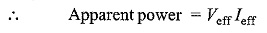In Eq. 6.10, the average power depends on the value of cos θ, this is called the power factor of the circuit.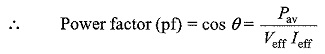Therefore, power factor is defined as the ratio of average power to the apparent power, whereas apparent power is the product of the effective values of the current and the voltage.

Power factor is also defined as the factor with which the volt amperes are to be multiplied to get true power in the circuit.

In the case of sinusoidal sources, the power factor is the cosine of the phase angle between voltage and current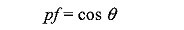As the phase angle between voltage and total current increases, the power factor decreases. The smaller the power factor, the smaller the power dissipation. The power factor varies from 0 to 1. For purely resistive circuits, the phase angle between voltage and current is zero, and hence the power factor is unity For purely reactive circuits, the phase angle between voltage and current is 90°, and hence the power factor is zero.

In an RC circuit, the power factor is referred to as leading power factor because the current leads the voltage. In an RL circuit, the power factor is referred to as lagging power factor because the current lags behind the voltage.

Scroll to Top